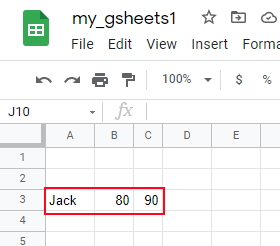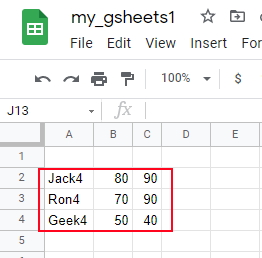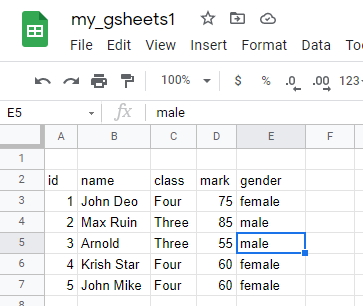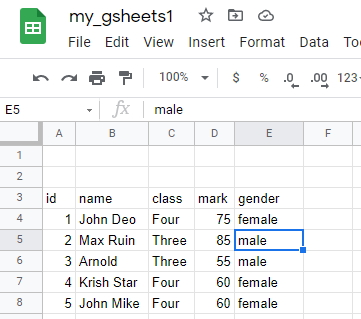Adding data from list, csv and excel file to Google sheet by append_table() in pygsheets

Data will be added to next row (or column ) of the table. ( Here row id or row number of the locations is not required )
``````append_table(values, start='A1', end=None, dimension='ROWS',
overwrite=False)``````
OptionsDetails
startStaring Point of top left cell ( 'A5')
endBottom right cell
dimensionThe way the value will be added, (Default : ROWS) ,ROWS or COLUMNS( See the example below ).
overwriteIf True the data will be overwritten
Connect to googel drive and open the file (more on connection & authorization)
``````import pygsheets
gc=pygsheets.authorize(service_account_file=path)
sh=gc.open('my_gsheets1')
wk1=sh``````
``````data1=['Jack',80,90]
wk1.append_table(data1,start='A3')``````## Using List

``````l1=[['Jack4',80,90],['Ron4',70,90],['Geek4',50,40]]
wk1.append_table(values=l1)``````

## Using a list with loop

``````l1=[['Jack4',80,90],['Ron4',70,90],['Geek4',50,40]]
for data in l1:
wk1.append_table(data,start='A2', end=None,
dimension='ROWS', overwrite=False)``````
By using loop we can add conditions and change the data based on required logic.## Using CSV file

Download the sample CSV file from here. We kept the file in our local system and updated the path below.
``````path="D:\\my_data\\student.csv" # sample CSV file, use your path
fob=open(path,)
for rec in fob:
student=rec.split(',')
print(student)
wk1.append_table(start='A2',values=student)``````In above code we have not used Pandas DataFrame. We can create the DataFrame by using Pandas read_csv() and then use the set_dataframe() to add data to google sheet.
``````import pandas as pd
path="D:\\my_data\\student.csv" # sample CSV file, use your path
wk1.set_dataframe(df,(3,1))# Place DataFrame from row 3 column 1``````## Using Excel file

``````import pandas as pd
path="D:\\my_data\\student.xlsx" # sample Excel file, use your path
wk1.set_dataframe(df,(3,1)) # Place DataFrame from row 3 column 1``````

## Using insert_row()

We should use insert_row() when we row number is available. At a perticular location the data can be added. This is the main difference between insert_row() and append_table()

Subscribe to our YouTube Channel here

## Subscribe

* indicates required
Subscribe to plus2netplus2net.com